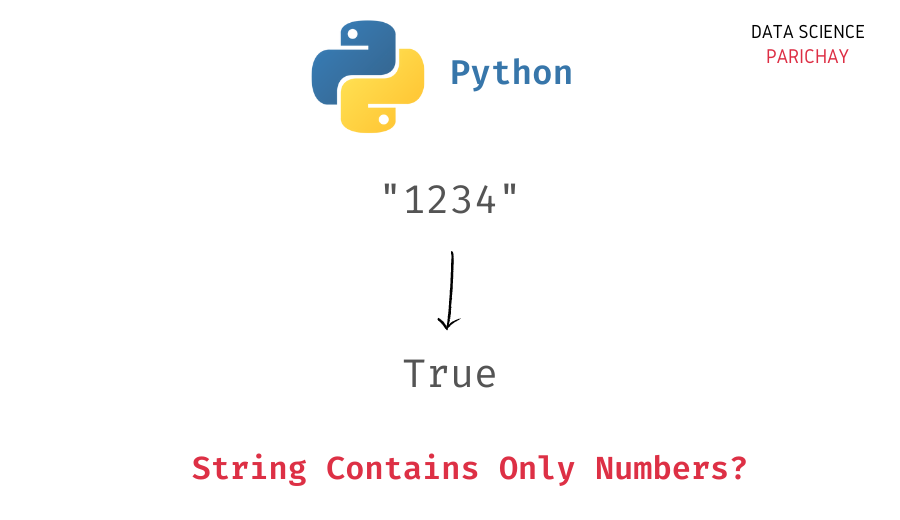# Python – Check if String Contains Only Numbers

In this tutorial, we will look at how to check whether a string contains only numbers or not in Python with the help of some examples.

You can use the string `isdigit()` function in Python to check whether a string only contains numbers or not. The following is the syntax –

```# check if string s contains only numbers
s.isdigit()```

It returns `True` if all the characters in the string are digits and `False` otherwise. (Note that this will return `False` if you use it on an empty string).

Let’s look at an example to see the usage of the above function.

```# create a string
s = "127"
# check if it only contains numbers
print(s.isdigit())```

Output:

`True`

You can see that we get `True` as the output since the string, s contained only numerical characters.

Let’s look at another example. This time, with a string where not all characters are numbers.

```# create a string
s = "127a"
# check if it only contains numbers
print(s.isdigit())```

Output:

📚 Data Science Programs By Skill Level

Introductory

Intermediate ⭐⭐⭐

🔎 Find Data Science Programs 👨‍💻 111,889 already enrolled

Disclaimer: Data Science Parichay is reader supported. When you purchase a course through a link on this site, we may earn a small commission at no additional cost to you. Earned commissions help support this website and its team of writers.

`False`

We get `False` as the output.

Note that the string `isdigit()` method will return `False` if it does not find all the characters to be numeric. This can be an issue if you’re using it on –

For example, we get `False` as the output, if we use the `isdigit()` function on negative numbers in a string.

```# create a string
s = "-127"
# check if it only contains numbers
print(s.isdigit())```

Output:

`False`

A workaround you can do is to use the string `lstrip()` function to remove the `-` sign from the starting of the string (if they are present) and then apply the string `isdigit()` function.

```# create a string
s = "-127"
# check if it only contains numbers including negative numbers
print(s.lstrip('-').isdigit())```

Output:

`True`

We now get `True` as the output.

Be careful about what you really want to use the `isdigit()` function for.

For example, if you want to check if all the characters in the string are digits or not, then it’s your perfect solution. But, if you want to check whether a string is a positive or a negative integer then be mindful of the `-` sign in the beginning.

What if your string contains a decimal point with numbers on either side? Using the `isdigit()` function on the entire string will return `False` as the string does not contain all the characters as digits.

```# create a string
s = "127.53"
# check if it only contains numbers
print(s.isdigit())```

Output:

`False`

To check if the string contains only numbers irrespective of the decimal point. You can use the following code.

```# create a string
s = "127.53"
# check if it only contains numbers irrespective of decimal point
ls = s.split(".")
print(all(n.isdigit() for n in ls) and len(ls) <= 2)```

Output:

`True`

Here we split the string on the decimal point and return `True` only if all the parts are digits and if there’s at a maximum only one decimal point in the string.

We can now combine our workarounds for negative and decimal numbers together to get a more robust check on whether a string is numerical or not irrespective of the presence of a negative sign or a decimal point.

```# create a string
s = "-127.53"
# check if it only contains numbers irrespective of negative sign and decimal point
ls = s.lstrip("-").split(".")
print(all(n.isdigit() for n in ls) and len(ls) <= 2)```

Output:

`True`

Again, if your objective is to check whether characters are digits or not, using the `isdigit()` function directly will suffice your requirement.

You might also be interested in –

•